﻿ SQL: Number of orders booked for each day

# SQL Exercise: Number of orders booked for each day

## SQL Formatting Output: Exercise-2 with Solution

From the following table, write a SQL query to find the number of orders booked for each day. Return the result in a format like "For 2001-10-10 there are 15 orders".

Sample table: orders

Sample Solution:

``````SELECT ' For',ord_date,',there are',
COUNT (ord_no),'orders.'
FROM orders
GROUP BY ord_date;``````

Output of the Query:

```?column?	ord_date	?column?	count	?column?
For		2012-04-25	,there are	1	orders.
For		2012-06-27	,there are	1	orders.
For		2012-07-27	,there are	1	orders.
For		2012-08-17	,there are	2	orders.
For		2012-09-10	,there are	3	orders.
For		2012-10-05	,there are	2	orders.
For		2012-10-10	,there are	2	orders.
```

Code Explanation:

The query in SQL retrieves data from the 'orders' table and returns the information: The string "For", the column ord_date, the string ",there are", the count of the column ord_no, calculated using the COUNT function and grouped by ord_date, and the string "orders."
In the end, the final result that displays the message "For (order_date), there are (count of order_no) orders." for each unique order_date value.

Relational Algebra Expression: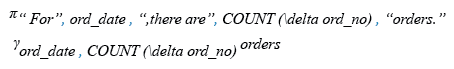Relational Algebra Tree: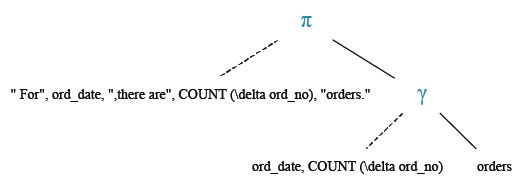Explanation :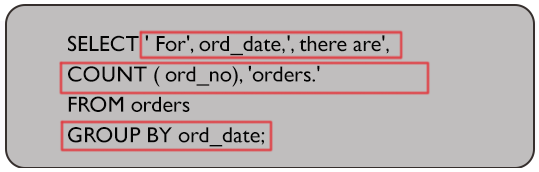Visual presentation :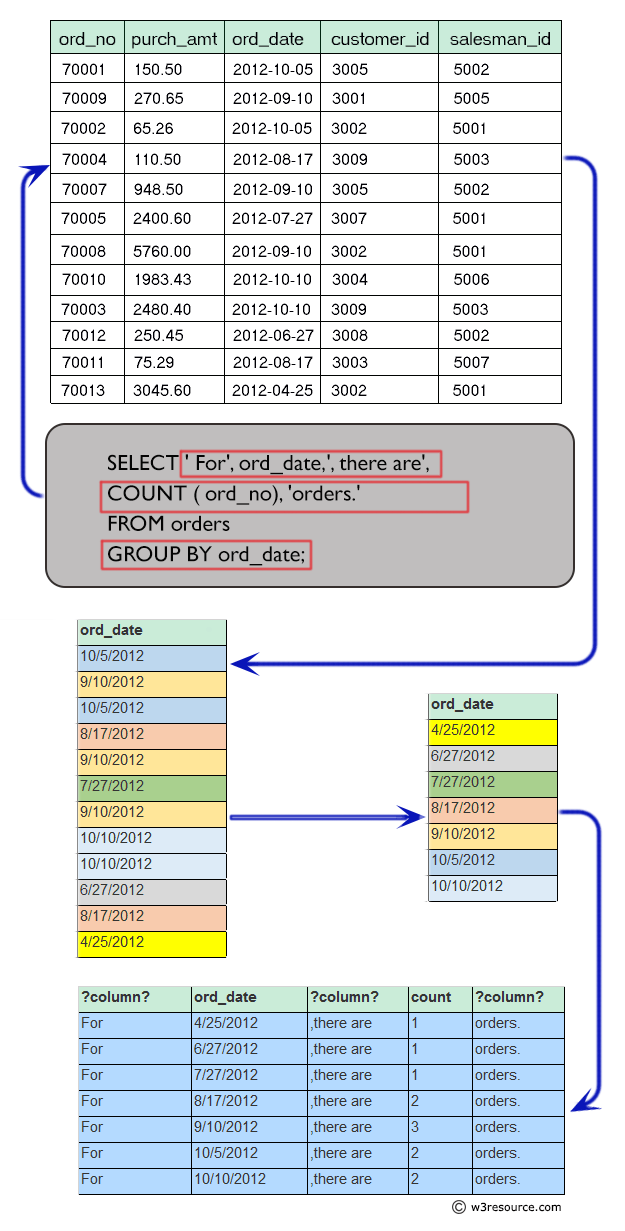## Query Visualization:

Duration: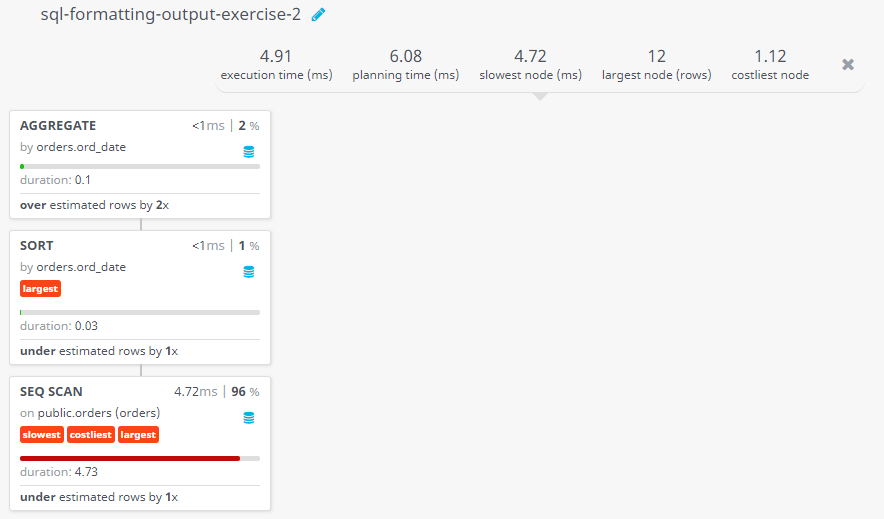Rows: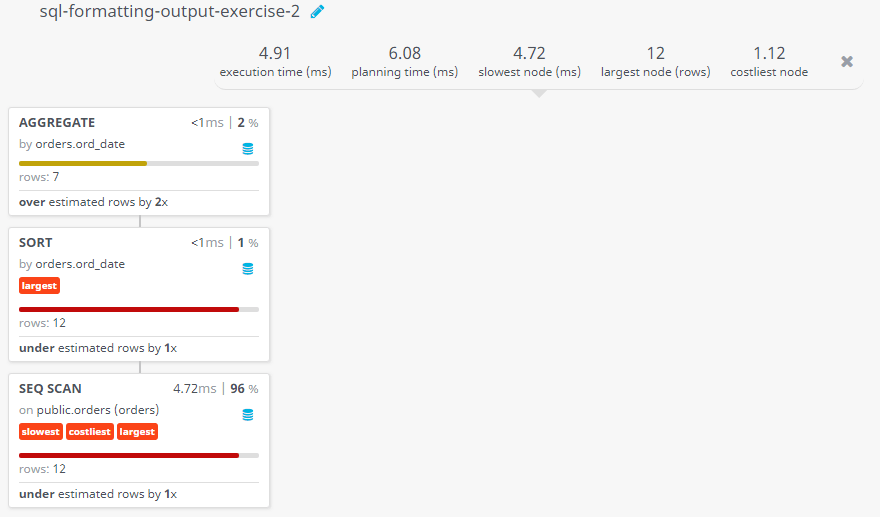Cost: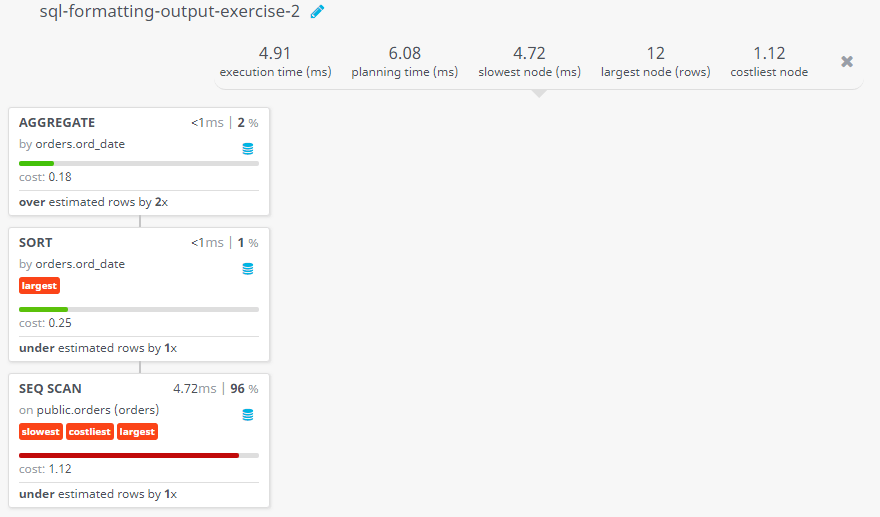Have another way to solve this solution? Contribute your code (and comments) through Disqus.

Previous SQL Exercise: Salesmen commission with the percent sign (%).
Next SQL Exercise: Display orders after sorting orders by order number.

What is the difficulty level of this exercise?

Test your Programming skills with w3resource's quiz.

﻿